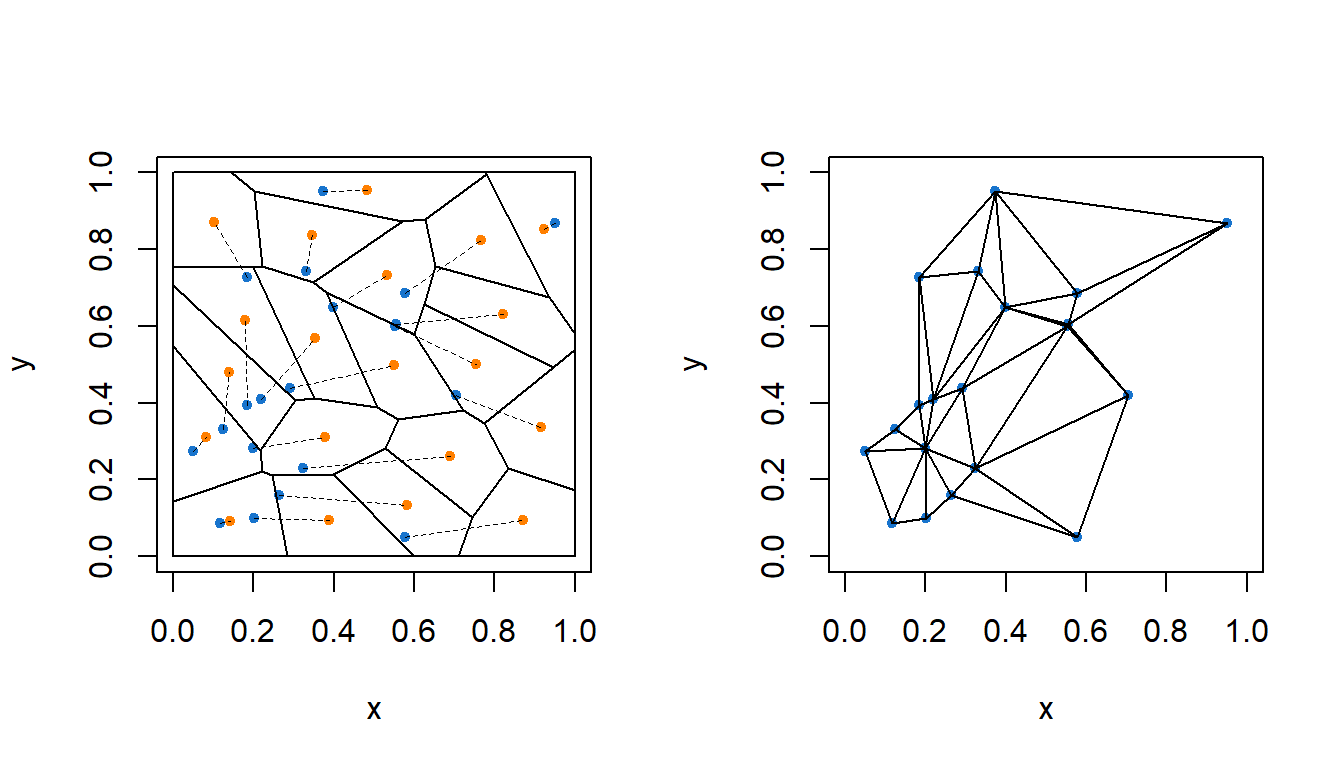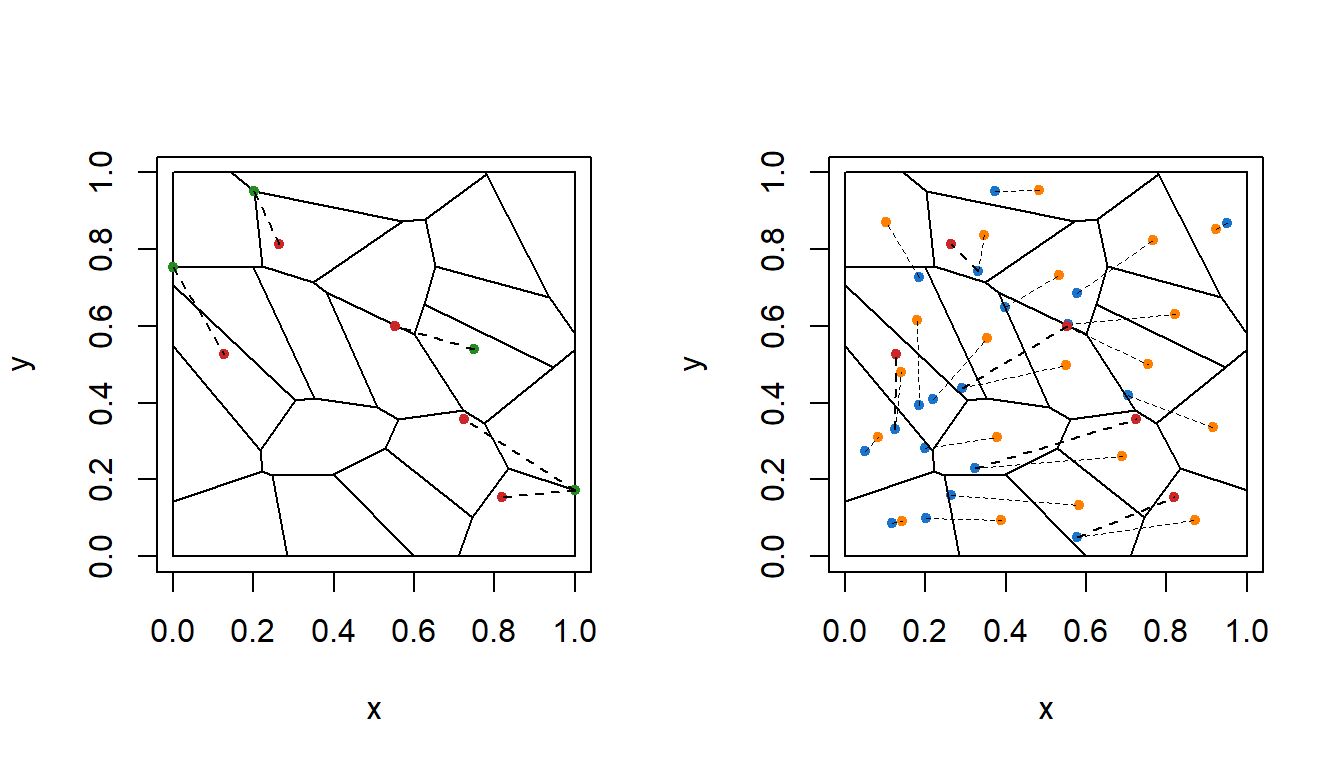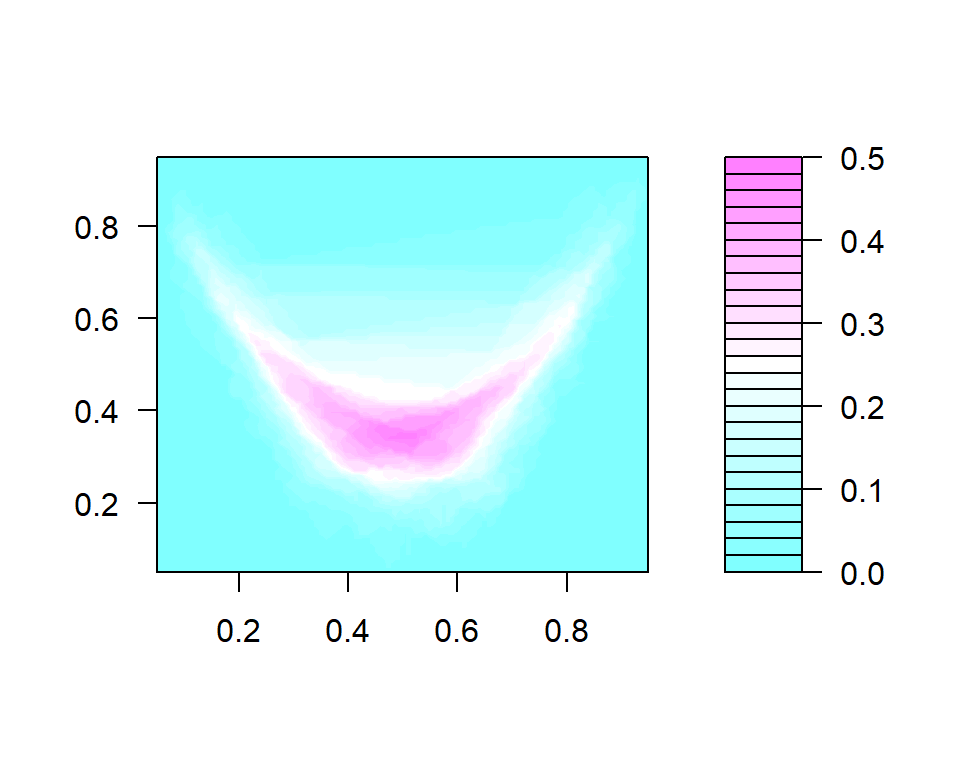# testOTM Vignette

#### 29 November 2019

testOTM is an R package that computes multivariate ranks and quantiles defined through the theory of optimal transportation. It also provides several applications of these statistics, most notably a method for two-sample multivariate goodness-of-fit testing.

## Introduction

As mentioned, the core of the ideas used by this package is optimal transportation: given Borel (probability) measures $$\mu$$ (source) and $$\nu$$ (target), whose supports are $$\mathcal{X}\subset\mathbb{R}^d$$ (which we assume to be convex and compact) and $$\mathcal{Y}\subset\mathbb{R}^d$$ respectively, a map $$T\colon\mathcal{X}\to\mathcal{Y}$$ attaining $\inf_{T: T\mathbin{\#}\mu=\nu}\int_{\mathcal{X}}\left\lVert x-T(x)\right\rVert_2^2\,d\mu(x)$ is called the ($$L_2$$-) optimal transport map, where the notation $$T\mathbin{\#}\mu=\nu$$ means $$\mu(T^{-1}(B))=\nu(B)$$ for all Borel $$B\subset\mathcal{Y}$$ (thus we say $$T$$ transports $$\mu$$ to $$\nu$$). When $$\nu$$ is univariate and $$\mu\sim U[0,1]$$, the map $$T$$ is an analog of the one-dimensional quantile function of $$\nu$$. Thus, by substituting $$\mu\sim U[0,1]^d$$ when $$\nu$$ is in $$\mathbb{R}^d$$, we are able to generalize the notion of quantiles (and ranks), see e.g. (Chernozhukov et al. 2017; Ghosal and Sen 2019) for more detail.

In application, we do not usually know the target measure $$\nu$$, but rather have a finite sample $$\{X_i\}_{i=1}^n\stackrel{\text{i.i.d.}}{\sim}\nu$$. We thus replace the unknown $$\nu$$ by its empirical distribution $$\hat{\nu}_n$$, which satisfies $\hat{\nu}_n(B)=\frac{1}{n}\sum_{i=1}^n\delta_{X_i}(B)$ for all Borel $$B\subset\mathcal{Y}$$. Accordingly, we define the empirical quantile function $$\hat{Q}_n$$ to be the solution of the optimal transport problem when $$\hat{\nu}_n$$ is used instead of $$\nu$$.

An important result, known as Brenier-McCann’s Theorem (Brenier 1991; McCann 1995), implies that there exists a piecewise affine function $$\hat{\psi}_n$$ such that $$\hat{Q}_n=\nabla\hat{\psi}_n$$. Since the rank function in 1D is the inverse of the quantile map, the multivariate empirical rank function can be defined as $\hat{R}_n(\mathbf{x})=\operatorname*{arg\,min}_{\mathbf{y}\in\mathcal{Y}}\{\mathbf{x}^\top\mathbf{y}-\hat{\psi}_n(\mathbf{y})\},$ and it can be computed through linear programming. This definition is justified by the inversion rule of sub-gradient: $\mathbf{x}\in\partial\hat{\psi}_n(\mathbf{y})\iff\mathbf{y}\in\partial\hat{\psi}_n\hspace{-5pt}{}^\ast(\mathbf{x}),$ where $$\hat{\psi}_n\hspace{-5pt}{}^\ast$$ is the Legendre-Fenchel dual of $$\hat{\psi}_n$$.

## Visualizing the Transport Map, Rank, and Quantiles

When the source measure $$\mu$$ is absolutely continuous and the target measure $$\nu$$ is discrete, the optimal transport problem is said to be semi-discrete. The solution in this case turns out to have a nice geometric interpretation: it is a partition of $$\mathcal{X}$$ by convex sets (cells) which correspond to $$X_i$$’s (seeds), and every element in the cells is tranported to the corresponding seeds. This partition is called the (restricted) power diagram or the Laguerre diagram (which in fact is a generalization of the Voronoi diagram), see (Aurenhammer 1987) for more information.

Efficient algorithms for solving the semi-discrete optimal transport problem in 2D/3D have been proposed in (Lévy and Schwindt 2018), and are implemented in the Geogram library. testOTM provides a wrapper to Geogram to solve the specific problems when $$\mu$$ equals $$U[0,1]^2$$ or $$U[0,1]^3$$.

In the following example, we demonstrate how to compute and visualize the optimal transport map from $$U[0,1]^2$$ to a (scaled) bivariate Gaussian sample $$\{X_i\}_{i=1}^{20}$$:

# generate bivariate normal sample
p = 2
n = 20
Sigma = matrix(c(3, 1, 1, 3), p, p)
eS = eigen(Sigma, symmetric = TRUE)
X = t(eS$vectors %*% diag(sqrt(pmax(eS$values, 0)), p) %*% matrix(rnorm(p * n), p))

# compute the optimal transport map from U[0, 1]^2 to X
# notice that X will be automatically scaled into [0, 1] range
# tos is an abbreviation for Transport Optimal Semi-discret
X.OTM = tos.fit(X)

# plot the restricted Voronoi diagram and the restricted Delaunay triangulation
oldpar = par(mfrow = c(1, 2))
plot(X.OTM, which = "Both", draw.center = T, draw.map = T)par(oldpar)

The two plots being produced are the restricted Voronoi diagram (RVD) and the restricted Delaunay triangulation (RDT). RVD is the partition we mentioned above, where each polygon (cell) corresponds to one datum (blue point), and any point within that cell will be transported to the datum by the optimal transport map. The orange points are the centroids of the cells. By setting draw.map = T, dashed lines will be drawn between the centroids and the data to indicate the correspondence. RDT, on the other hand, is the dual graph of the RVD and has many computational applications, see for example (Cheng, Dey, and Shewchuk 2013; Toth, O’Rourke, and Goodman 2017) for more information.

Now with a fitted tos.2d object available, we can use it to compute the 2D empirical ranks and quantiles. The following snippet shows how to do so for some arbitrary points $$\{Y_i\}_{i=1}^{5}$$:

# choose some arbitrary points
Y = matrix(c(3.2, -0.9,
-1.2, 1.8,
4.1, -2.1,
-2.5, 0.1,
0, 0), ncol = 2, byrow = T)
Y[5, ] = X[1, ] # take one point from data
R = tos.rank(X.OTM, Y) # compute ranks
Q = tos.quantile(X.OTM, Y) # compute quantiles

# to visualize ranks and quantiles, we first apply the scaling of X on Y
Y = scale(Y, X.OTM$Location, X.OTM$Scale)
Y = Y * diff(range(X.OTM$Data)) + min(X.OTM$Data)

# plot ranks
oldpar = par(mfrow = c(1, 2))
plot(X.OTM, which = "RVD", draw.center = F, draw.map = F, draw.data = F)
points(Y, col = "firebrick3", pch = 20)
points(R$Rank, col = "forestgreen", pch = 20) segments(R$Rank[, 1], R$Rank[, 2], Y[, 1], Y[, 2], lty = 2) # plot quantiles plot(X.OTM, which = "RVD", draw.center = T, draw.map = T, draw.data = T) points(Y, col = "firebrick3", pch = 20) segments(Q$Quantiles[, 1], Q$Quantiles[, 2], Y[, 1], Y[, 2], lty = 2)par(oldpar) The plot on the left shows the query points (red) and their corresponding ranks (green). By the nature of their definition, ranks are always vertices of some cells in the RVD, except when the query is one of the data point $$X_i$$, in which case $$\hat{R}_n$$ is not uniquely defined and can be any point in the closure of the cell corresponds to $$X_i$$. By default, tos.rank will sample a point uniformly from that cell. The plot on the right, meanwhile, shows the quantiles of the query points. As pointed out earlier, the query points will be map to the data points that correspond to the cells they are in. The similar visualization procedure can be performed for 3D data sets as well. testOTM supports both interactive (via package rgl) and non-interactive (via package plot3D) plotting of the RVD and the RDT. The following example shows the optimal transport map (RVD) between $$U[0,1]^3$$ and a (scaled) trivariate Gaussian sample: # generate trivariate normal data p = 3 n = 10 Sigma = matrix(c(2, 1, 1, 1, 2, 1, 1, 1, 2), p, p) eS = eigen(Sigma, symmetric = TRUE) X = t(eS$vectors %*% diag(sqrt(pmax(eS$values, 0)), p) %*% matrix(rnorm(p * n), p)) # compute the optimal transport map from U[0, 1]^3 to the data X.OTM = tos.fit(X) #> o-[OTM ] In RVD (centroids)... #> o-[RVD ] Elapsed time: 0 s # plot the restricted Voronoi diagram and the restricted Delaunay triangulation plot(X.OTM, inter = TRUE, which = "RVD", draw.center = T, draw.map = T) # embed the plot into the current document rglwidget(elementId = "plot3drgl", height = 500, width = 500) Back to Top ## Nonparametric Testing With multivariate ranks and quantiles defined, we can use them to construct nonparametric test statistics for various uses. One such application is the goodness-of-fit testing: given independent $$\{X_i\}_{i=1}^n\sim\nu_X$$ and $$\{Y_i\}_{i=1}^m\sim\nu_Y$$, we want to test $H_0\colon\nu_X=\nu_Y\qquad\text{versus}\qquad H_1\colon\nu_X\neq\nu_Y.$ Function tos.gof.test implements the statistic (for 2D and 3D) proposed in (Ghosal and Sen 2019) for this test: $T_{X,Y}=\int_{[0,1]^d}\left\lVert\hat{R}_{X,Y}(\hat{Q}_{X}(u))-\hat{R}_{X,Y}(\hat{Q}_{Y}(u))\right\rVert_2^2\,d\mu(u),$ where $$\mu=U[0,1]^d$$. Under the null hypothesis, $$T_{X,Y}$$ is expected to be small so we reject $$H_0$$ if $$T_{X,Y}$$ exceeds certain critical value. However, since the null distribution of $$T_{X,Y}$$ has not been characterized, we compute the $$p$$-value through permutations instead. In addition, the integral in the definition of $$T_{X,Y}$$ is evaluated through quasi-Monte Carlo, and by default we use a Sobol sequence of length $$10000$$. The following example showcases a goodness-of-fit test given $$H_0$$ false. For simplicity we set the number of permutations to $$100$$, in practice this parameter is usually set to $$500$$, $$1000$$, or $$1500$$. # simulate data, H_0 false p = 2 n = 100 Sigma1 = matrix(c(3, 1, 1, 3), p, p) eS = eigen(Sigma1, symmetric = TRUE) X = t(eS$vectors %*% diag(sqrt(pmax(eS$values, 0)), p) %*% matrix(rnorm(p * n), p)) Sigma2 = matrix(c(5, 3, 3, 2), p, p) eS = eigen(Sigma2, symmetric = TRUE) Y = t(eS$vectors %*% diag(sqrt(pmax(eS$values, 0)), p) %*% matrix(rnorm(p * n), p)) # two sample goodness-of-fit test GoF = tos.gof.test(X, Y, n.perm = 100) #> Consider setting n.perm to a larger value, otherwise the permutation p-value may not be reliable. GoF #>$perm.stats
#>    0.05547294 0.01362790 0.02020353 0.02025701 0.02222902 0.01547936
#>    0.01775848 0.01510168 0.02076719 0.01271096 0.02889391 0.01589894
#>   0.01733697 0.02083321 0.01310197 0.01815264 0.01476966 0.01402921
#>   0.04092426 0.01297677 0.02086594 0.01922223 0.01627245 0.01778115
#>   0.01559354 0.02069145 0.01589480 0.01770116 0.01783689 0.01821656
#>   0.01455673 0.02269634 0.01937998 0.01361806 0.01664510 0.01908505
#>   0.01742552 0.02645806 0.02266787 0.01691875 0.01683172 0.01768728
#>   0.01511479 0.01708146 0.01722143 0.01275648 0.01964849 0.02114601
#>   0.02529509 0.01726285 0.01492604 0.01775032 0.01606573 0.01908499
#>   0.01877251 0.02560740 0.01515603 0.01745057 0.01483496 0.01969811
#>   0.01544080 0.01825925 0.02120513 0.01411049 0.01752653 0.01612723
#>   0.01568712 0.02220282 0.01356071 0.01963889 0.01731658 0.01644406
#>   0.02146766 0.01445971 0.02676911 0.01803858 0.02336171 0.03397531
#>   0.02224157 0.02315803 0.01806431 0.01927216 0.02286131 0.01920470
#>   0.03045460 0.02080260 0.01981565 0.01389055 0.02064275 0.01999544
#>   0.01805560 0.01490642 0.04178384 0.01436522 0.01584841 0.01928399
#>   0.01698221 0.01437074 0.02017172 0.01729559 0.02056135
#>
#> $p.value #>  0.00990099 Another useful application of the multivariate ranks and quantiles in nonparametric testing is the test of independence: given samples $$\{Z_i=(X_i, Y_i)\}\sim\nu$$, where $$X_i\sim\nu_X$$ and $$Y_i\sim\nu_Y$$, we’d like to test $H_0\colon\nu=\nu_X\otimes\nu_Y\qquad\text{versus}\qquad H_1\colon\nu\neq\nu_X\otimes\nu_Y.$ A test statistic for this is presented in (Ghosal and Sen 2019) and reduced to the following when both $$X_i$$ and $$Y_i$$ are univariate: $T_n=\frac{1}{n}\sum_{i=1}^n\left\lVert\hat{R}_{Z}(Z_i)-\tilde{R}_{Z}(Z_i)\right\rVert_2^2,$ where $$\tilde{R}_{Z}=(\hat{R}_{X},\hat{R}_{Y})$$ is simply the joining of the usual 1D ranks of $$X$$ and $$Y$$. The following snippet demonstrates how to perform this test with tos.dep.test: # simulate data, H_0 false p = 2 n = 100 Sigma = matrix(c(5, 3, 3, 2), p, p) eS = eigen(Sigma, symmetric = TRUE) Z = t(eS$vectors %*% diag(sqrt(pmax(eS$values, 0)), p) %*% matrix(rnorm(p * n), p)) X = Z[, 1] Y = Z[, 2] # test of independence Dep = tos.dep.test(X, Y, n.perm = 100) #> Consider setting n.perm to a larger value, otherwise the permutation p-value may not be reliable. Dep #>$perm.stats
#>    0.073955649 0.006177616 0.004226963 0.005793903 0.004320816 0.004690612
#>    0.006733018 0.004609397 0.005080074 0.005418031 0.004523353 0.004597243
#>   0.004373057 0.005121912 0.004259679 0.005663628 0.006680496 0.004720457
#>   0.006057038 0.004063153 0.008997803 0.004553653 0.004825803 0.008291555
#>   0.004868246 0.005699579 0.004414302 0.004951877 0.006407556 0.005093551
#>   0.006193386 0.007394195 0.004538507 0.004762306 0.005241381 0.004534415
#>   0.004728670 0.004982014 0.004577619 0.004352718 0.006342457 0.004682291
#>   0.003845522 0.004739927 0.006385566 0.006002056 0.003887186 0.005492832
#>   0.006831751 0.005825017 0.006291243 0.004359714 0.004459156 0.004908913
#>   0.004680220 0.005166086 0.005809388 0.004913375 0.005618704 0.004197121
#>   0.004603629 0.005548422 0.004877512 0.005071068 0.007974345 0.004657016
#>   0.004846608 0.005564945 0.006059724 0.005657148 0.005201459 0.009355856
#>   0.006210806 0.008932093 0.005189654 0.005117989 0.004451350 0.005415067
#>   0.005977587 0.006200871 0.004837297 0.007735402 0.004102528 0.006951765
#>   0.004590252 0.005730797 0.006211346 0.005109265 0.004776707 0.005540336
#>   0.005480806 0.003912989 0.005235286 0.004336223 0.004186718 0.004405226
#>   0.005264587 0.004075163 0.004923141 0.006744831 0.004581869
#>
#> \$p.value
#>  0.00990099

## Statistical Depth

The statistical depth is a measurement of the center-outward ordering of points in the support of a distribution, and it can be used to generalize some univariate notions, e.g. the median, to multivariate. Following suggestion of (Chernozhukov et al. 2017), we compute the sample depth of $$\{X_i\}_{i=1}^n\sim\nu$$ in $$\mathbb{R}^d$$ as $\hat{D}_n(\mathbf{x})=\frac{1}{2}-\left\lVert\hat{R}_{n}(\mathbf{x})-\frac{1}{2}\mathbf{1}_d\right\rVert_\infty.$ The following example shows the sample depths of the banana-shaped data presented in (Chernozhukov et al. 2017):

# simulate banana-shaped data
n = 1000
x = runif(n, -1, 1)
phi = runif(n, 0, 2 * pi)
z = runif(n, 0, 1)
r = 0.2 * z * (1 + (1 - abs(x)) / 2)
X = cbind(x + r * cos(phi), x^2 + r * sin(phi))

# fit a transport map
X.OTM = tos.fit(X)

# set a grid to evaluate the depth, so that we can plot them
k = 100
q = seq(0.05, 0.95, length.out = k)
Q = as.matrix(expand.grid(q, q))

# compute depth, then plot its heatmap
D = tos.depth(X.OTM, Q, scale = F)
filled.contour(q, q, matrix(D, k, k), color.palette = function(n) cm.colors(n))## Reference

Aurenhammer, F. 1987. “Power Diagrams: Properties, Algorithms and Applications.” SIAM Journal on Computing 16 (1): 78–96. https://doi.org/10.1137/0216006.

Brenier, Yann. 1991. “Polar Factorization and Monotone Rearrangement of Vector-Valued Functions.” Communications on Pure and Applied Mathematics 44 (4): 375–417. https://doi.org/10.1002/cpa.3160440402.

Cheng, Siu-Wing, Tamal Krishna Dey, and Jonathan Richard Shewchuk. 2013. Delaunay Mesh Generation. Chapman; Hall/CRC.

Chernozhukov, Victor, Alfred Galichon, Marc Hallin, and Marc Henry. 2017. “Monge–Kantorovich Depth, Quantiles, Ranks and Signs.” The Annals of Statistics 45 (1): 223–56. https://doi.org/10.1214/16-AOS1450.

Ghosal, Promit, and Bodhisattva Sen. 2019. “Multivariate Ranks and Quantiles Using Optimal Transportation and Applications to Goodness-of-Fit Testing.” http://arxiv.org/abs/1905.05340.

Lévy, Bruno, and Erica L. Schwindt. 2018. “Notions of Optimal Transport Theory and How to Implement Them on a Computer.” Computers & Graphics 72: 135–48. https://doi.org/10.1016/j.cag.2018.01.009.

McCann, Robert J. 1995. “Existence and Uniqueness of Monotone Measure-Preserving Maps.” Duke Math. J. 80 (2): 309–23. https://doi.org/10.1215/S0012-7094-95-08013-2.

Toth, Csaba D., Joseph O’Rourke, and Jacob E. Goodman, eds. 2017. Handbook of Discrete and Computational Geometry. 3rd ed. Chapman; Hall/CRC.